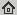# Non-smooth non-convex Bregman minimization: unification and new algorithms

Journal of Optimization Theory and Applications, 2019
Abstract: We propose a unifying algorithm for non-smooth non-convex optimization. The algorithm approximates the objective function by a convex model function and finds an approximate (Bregman) proximal point of the convex model. This approximate minimizer of the model function yields a descent direction, along which the next iterate is found. Complemented with an Armijo-like line search strategy, we obtain a flexible algorithm for which we prove (subsequential) convergence to a stationary point under weak assumptions on the growth of the model function error. Special instances of the algorithm with a Euclidean distance function are, for example, Gradient Descent, Forward{Backward Splitting, ProxDescent, without the common requirement of a \Lipschitz continuous gradient". In addition, we consider a broad class of Bregman distance functions (generated by Legendre functions), replacing the Euclidean distance. The algorithm has a wide range of applications including many linear and non-linear inverse problems in signal/image processing and machine learning.

## BibTex reference

```@Article{OB19,
author       = "P. Ochs and J. Fadili and T. Brox",
title        = "Non-smooth non-convex Bregman minimization: unification and new algorithms",
journal      = "Journal of Optimization Theory and Applications",
month        = " ",
year         = "2019",
url          = "http://lmb.informatik.uni-freiburg.de/Publications/2019/OB19"
}
```